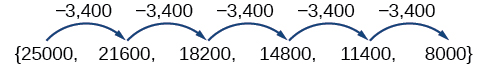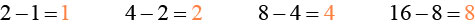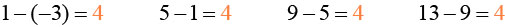# 11.2 Arithmetic sequences

 Page 1 / 8
In this section, you will:
• Find the common difference for an arithmetic sequence.
• Write terms of an arithmetic sequence.
• Use a recursive formula for an arithmetic sequence.
• Use an explicit formula for an arithmetic sequence.

Companies often make large purchases, such as computers and vehicles, for business use. The book-value of these supplies decreases each year for tax purposes. This decrease in value is called depreciation. One method of calculating depreciation is straight-line depreciation, in which the value of the asset decreases by the same amount each year.

As an example, consider a woman who starts a small contracting business. She purchases a new truck for $25,000. After five years, she estimates that she will be able to sell the truck for$8,000. The loss in value of the truck will therefore be $17,000, which is$3,400 per year for five years. The truck will be worth $21,600 after the first year;$18,200 after two years; $14,800 after three years;$11,400 after four years; and \$8,000 at the end of five years. In this section, we will consider specific kinds of sequences that will allow us to calculate depreciation, such as the truck’s value.

## Finding common differences

The values of the truck in the example are said to form an arithmetic sequence because they change by a constant amount each year. Each term increases or decreases by the same constant value called the common difference of the sequence. For this sequence, the common difference is –3,400.The sequence below is another example of an arithmetic sequence. In this case, the constant difference is 3. You can choose any term    of the sequence    , and add 3 to find the subsequent term.## Arithmetic sequence

An arithmetic sequence    is a sequence that has the property that the difference between any two consecutive terms is a constant. This constant is called the common difference    . If ${a}_{1}$ is the first term of an arithmetic sequence and $d$ is the common difference, the sequence will be:

$\left\{{a}_{n}\right\}=\left\{{a}_{1},{a}_{1}+d,{a}_{1}+2d,{a}_{1}+3d,...\right\}$

## Finding common differences

Is each sequence arithmetic? If so, find the common difference.

1. $\left\{1,2,4,8,16,...\right\}$
2. $\left\{-3,1,5,9,13,...\right\}$

Subtract each term from the subsequent term to determine whether a common difference exists.

1. The sequence is not arithmetic because there is no common difference.2. The sequence is arithmetic because there is a common difference. The common difference is 4.If we are told that a sequence is arithmetic, do we have to subtract every term from the following term to find the common difference?

No. If we know that the sequence is arithmetic, we can choose any one term in the sequence, and subtract it from the subsequent term to find the common difference.

Is the given sequence arithmetic? If so, find the common difference.

The sequence is arithmetic. The common difference is $–2.$

Is the given sequence arithmetic? If so, find the common difference.

The sequence is not arithmetic because $3-1\ne 6-3.$

## Writing terms of arithmetic sequences

Now that we can recognize an arithmetic sequence, we will find the terms if we are given the first term and the common difference. The terms can be found by beginning with the first term and adding the common difference repeatedly. In addition, any term can also be found by plugging in the values of $n$ and $d$ into formula below.

a colony of bacteria is growing exponentially doubling in size every 100 minutes. how much minutes will it take for the colony of bacteria to triple in size
what is the importance knowing the graph of circular functions?
can get some help basic precalculus
What do you need help with?
Andrew
how to convert general to standard form with not perfect trinomial
can get some help inverse function
ismail
Rectangle coordinate
how to find for x
it depends on the equation
Robert
whats a domain
The domain of a function is the set of all input on which the function is defined. For example all real numbers are the Domain of any Polynomial function.
Spiro
foci (–7,–17) and (–7,17), the absolute value of the differenceof the distances of any point from the foci is 24.
difference between calculus and pre calculus?
give me an example of a problem so that I can practice answering
x³+y³+z³=42
Robert
dont forget the cube in each variable ;)
Robert
of she solves that, well ... then she has a lot of computational force under her command ....
Walter
what is a function?
I want to learn about the law of exponent
explain this
what is functions?
A mathematical relation such that every input has only one out.
Spiro
yes..it is a relationo of orders pairs of sets one or more input that leads to a exactly one output.
Mubita
Is a rule that assigns to each element X in a set A exactly one element, called F(x), in a set B.
RichieRich
If the plane intersects the cone (either above or below) horizontally, what figure will be created?ByBy OpenStaxBy OpenStaxBy Tess ArmstrongBy Cath YuBy Yasser IbrahimBy Saylor FoundationBy Dan ArielyBy OpenStaxBy OpenStaxBy Ellie Banfield